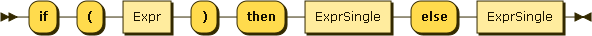### Conditional expressions

Figure 49. IfExprA conditional expressions allows you to pick one or another value depending on a boolean value.

Example 96. A conditional expression

```if (1 + 1 eq 2) then { "foo" : "yes" } else { "foo" : "false" }
```

Result (run with Zorba): { "foo" : "yes" }

The behavior of the expression inside the if is similar to that of logical operations (two-valued logics), meaning that non-boolean values get converted to a boolean.

Example 97. A conditional expression

```if (null) then { "foo" : "yes" } else { "foo" : "no" }
```

Result (run with Zorba): { "foo" : "no" }

Example 98. A conditional expression

```if (1) then { "foo" : "yes" } else { "foo" : "no" }
```

Result (run with Zorba): { "foo" : "yes" }

Example 99. A conditional expression

```if (0) then { "foo" : "yes" } else { "foo" : "no" }
```

Result (run with Zorba): { "foo" : "no" }

Example 100. A conditional expression

```if ("foo") then { "foo" : "yes" } else { "foo" : "no" }
```

Result (run with Zorba): { "foo" : "yes" }

Example 101. A conditional expression

```if ("") then { "foo" : "yes" } else { "foo" : "no" }
```

Result (run with Zorba): { "foo" : "no" }

Example 102. A conditional expression

```if (()) then { "foo" : "yes" } else { "foo" : "no" }
```

Result (run with Zorba): { "foo" : "no" }

Example 103. A conditional expression

```if (({ "foo" : "bar" }, [ 1, 2, 3, 4])) then { "foo" : "yes" } else { "foo" : "no" }
```

Result (run with Zorba): { "foo" : "yes" }

Note that the else clause is mandatory (but can be the empty sequence)

Example 104. A conditional expression

```if (1+1 eq 2) then { "foo" : "yes" } else ()
```

Result (run with Zorba): { "foo" : "yes" }